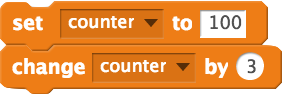Select Page

## Language Translation

### New Programming Languages

The goal of this homework is to introduce you to three new programming languages! We want to help you see that learning new programming languages will be easier now that you know Scratch! This activity will help you see connections across three other languages that you might have heard of. Pretty impressive, huh?

You’ve learned to do a lot with the programming language Scratch, so you’ll use that experience and your experience identifying patterns to learn these new languages. It is okay to guess!

Before we start, we want to know if you’ve heard of the programming languages we’ll be using in this assignment. For these questions, both answers are correct.

### Hello, my name is…

The code below is in four different languages: Scratch, C++, Java, and Python! Each of these scripts does exactly the same thing! In the boxes below, add one line of code to each script (Scratch, C++, Java, and Python) so that the script will say your first name.

## Scratch

Write out the words on the Scratch block that you want use at the end of the Scratch script below. For example, if you use this block:, write

`move 10 steps`

.## C++

```cout << "Hello";
cout << "my";
cout << "name";
cout << "is";
```

## Java

```System.out.print("Hello");
System.out.print("my");
System.out.print("name");
System.out.print("is");
```

## Python

```print "Hello"
print "my"
print "name"
print "is"
```

Look at the code above, and take a second to reflect on what each language has in common with the others. What similarities do you notice between C++ and Java? Between Java and Python? Are any parts of code commonly used between these pairs of languages? These questions are important for being able to apply your programming skills in other languages.

In all of the languages we’re looking at today (Scratch, C++, Java, and Python) there are shortcuts for adding a number to a variable. For each language, on the left we show the normal way to add 3 to the variable counter. On the right we show the shortcut.

### Scratch### C++

```int counter = 100;
counter = counter + 3;```
```int counter = 100;
counter += 3;```

### Java

```int counter = 100;
counter = counter + 3;```
```int counter = 100;
counter += 3;```

### Python

```counter = 100
counter = counter + 3```
```counter = 100
counter += 3```